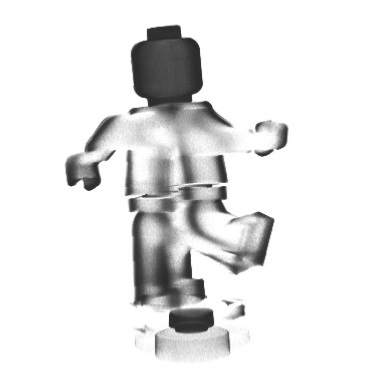# Students Project: Modeling X-ray tomography using low power lampsIn the occasion of celebrating 100 years of Radon transform, we were asked to model X-ray tomography experiments in a seminar room. The examples took place in the Computational Science Center (CSC) at the University of Vienna, Austria, under the supervision of Otmar Scherzer.

The tomographic system was replaced by an overhead projector, modeling the X-rays as light beams. The samples where rotated by a servomotor and for every rotation the overhead projector mapped the image of the object’s current position on the wall. The projections were recorded with a camera.

The aim of this project was to reconstruct objects from multiple projections. A Lego figure and a bottle, of the same size, were used as samples. The images correspond to the measured intensity of light passing through the object. Mathematically, this can be modeled considering Beer’s law. Then, under the assumption that light travels in straight light and is monochromatic we could compute the inverse Radon transform, in order to recover the geometry of the samples. The algorithm was implemented in Matlab, using the iradon function which is based on Filtered Back Projection Formula.

The output was a three dimensional matrix on the computer. One can imagine such an array as a cube consisting of many smaller cubes with values describing the opacity of the material in the specific part.

Then, we used a rendering program, coded by Axel Kittenberger (IT in CSC), to visualize the objects. The reconstruction of the small bottle is given in the left picture of Figure where the bright area corresponds to the inner part of the bottle. In the right picture, we see the reconstruction of the Lego figure. Since the object is not transparent the intensity of the light was almost zero inside, thus we could visualize only the boundary of the object

.

This project was carried out by Klaus Kastner, Romana Jezek and
Simone Altmann, all students in the Master program of applied mathematics and scientific computing in the University of Vienna. Klaus is interested in machine learning and Simone in stochastic analysis and dynamical systems.

References
T. G. Feeman, The Mathematics of Medical Imaging, Springer, 2010.